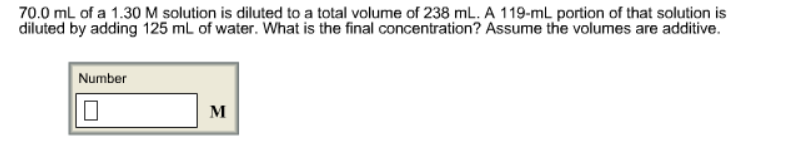# Problem: 70.0 mL of a 1.30 M solution is diluted to a total volume of 238 mL. A 119-mL portion of that solution is diluted by adding 125 mL of water. What is the final concentration? Assume the volumes are additive.

###### FREE Expert Solution
100% (214 ratings)###### Problem Details

70.0 mL of a 1.30 M solution is diluted to a total volume of 238 mL. A 119-mL portion of that solution is diluted by adding 125 mL of water. What is the final concentration? Assume the volumes are additive.